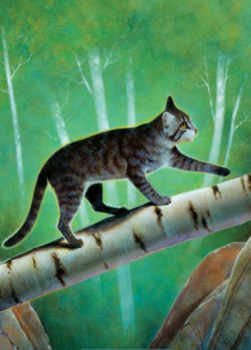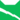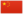# 炭心

Cinderheart《终极指南》雷族 武士雷族 巫医雷族 武士雷族 猫后雷族 武士雷族 猫后雷族 武士小炭、小煤 Cinderkit  小煤
 炭爪、煤爪 Cinderpaw  煤掌
 炭心 Cinderheart  煤心

Q3158：雷族母猫，自《新预言》系列登场的角色

## 细节

### 外貌

• 她是一只身材苗条、:66:24蓝色眼睛:10的烟:168灰色虎斑母猫。:猫物表她有着柔软的皮毛，:35头颅宽阔，以及一条长而光滑的:35刷状尾巴。:237
• 她最初被描述为“和炭毛一模一样”的暗灰色，:27但后来更变为烟灰色虎斑。:6

## 语录

—— 叶池和松鸦爪，关于炭爪，《暗河汹涌》正体中文版，第137-138页

—— 狮焰与炭心，《战声渐近》，第235页

—— 炭心与狮焰，《暗夜密语》，第114页

—— 炭心听说狮焰的能力后，《暗夜密语》，第141-143页

—— 炭心，在狮焰与风皮打斗后，《暗夜密语》正体中文版，第200页

—— 松鸦羽对炭心，《武士归来》，第198页

—— 狮焰与炭心，关于炭心的命运，《群星之战》，第243-244页

## 登场书目

• 黄昏战争 (Q143)[配角][首次登场🐱]
• 日落和平 (Q144)[配角]
• 预视力量 (Q152)[配角]
• 暗河汹涌 (Q153)[配角]
• 驱逐之战 (Q154)[配角]
• 天蚀遮月 (Q155)[配角]
• 暗夜长影 (Q156)[配角]
• 拂晓之光 (Q157)[配角]
• 第四学徒 (Q158)[配角]
• 战声渐近 (Q159)[配角]
• 暗夜密语 (Q160)[配角]
• 月光印记 (Q161)[配角]
• 武士归来 (Q162)[配角]
• 群星之战 (Q163)[配角]
• 学徒探索 (Q170)[对话提及]
• 雷影交加 (Q171)[配角]
• 天空破碎 (Q172)[配角]
• 极夜无光 (Q173)[配角]
• 烈焰焚河 (Q174)[配角]
• 风暴来袭 (Q175)[配角]
• 迷失群星 (Q3563)[配角]
• 静默冰融 (Q3579)[配角]
• 叠影重障 (Q3624)[配角]
• 暗由心生 (Q4183)[配角]
• 无星之地 (Q4385)[配角]
• 迷雾明光 (Q4621)[猫物闲角]
• 河翻浪涌 (Q4643)[配角]
• 黑莓星的风暴 (Q182)[配角]
• 虎心的阴影 (Q185)[猫物闲角]
• 鸦羽的拷问 (Q186)[配角]
• 松鼠飞的希冀 (Q3561)[配角]
• 灰条的誓言 (Q4057)[猫物闲角]
• 冬青叶的故事 (Q187)[配角]
• 雾星的征兆 (Q188)[猫物闲角]
• 叶池的祈愿 (Q191)[配角]
• 鸽翅的沉默 (Q192)[配角]
• 褐皮的族群 (Q3566)[猫物闲角]
• 黛西的至亲 (Q3629)[对话提及]
• 斑毛的叛变 (Q4337)[配角]
• 终极指南 (Q204)[配角]

1. 因放逐而离开

## 参考文献

这篇文章基于CC BY-SA 3.0许可使用了猫武士维基（英语）Cinderheart一文中的部分内容。
1. 《日落和平》第一章 (Q1048)
2. 极夜无光 (Q173)风暴来袭 (Q175)
3. 《日落和平》（简体中文）.未来出版社
4. 《预视力量》（简体中文）.未来出版社
5. 《预视力量》（简体中文）.中国少年儿童出版社
6. 烈焰焚河 (Q174)
7. 预视力量
8. 暗夜密语
9. 暗河汹涌
10. 群星之战
11. 艾琳·亨特访谈4
12. 战声渐近
13. 日落和平
0.0
0人评价
CXuesong
1

凯特就这样把转世的锅甩给了维琪……

5年